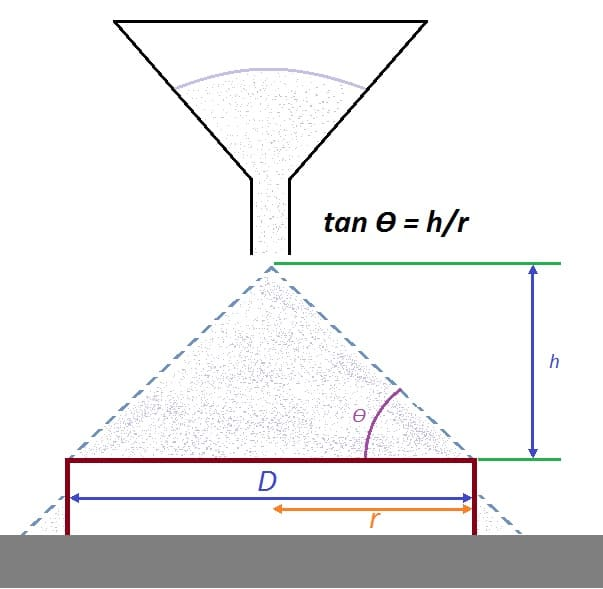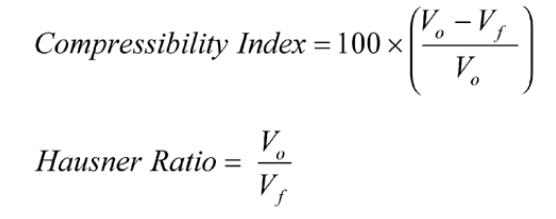# Simple methods to determine powder flow property

Most commonly used testing method to determine powder flow – Hausner ratio, Angle of repose, and Compressibility index

To get FREE Excel Sheet for calculation of powder flow property – Angle of repose, Compressibility index, and Hausner ratio, refer end section of the page.

## What are the most widely used testing methods to determine powder flow?

There are following four commonly used methods:
(1) Compressibility index or Hausner ratio
(2) Angle of repose
(3) Shear cell
(4) Flow rate through an orifice

## What is Angle of Repose?

The angle of repose is a simple technique for estimating the powder flow properties.

## How to determine Angle of Repose?

Step 1: Flow the powder through a funnel
Step 2: Powder should fall freely onto a fixed diameter base
Step 3: Measure height. Measure the diameter of the cone formed.

## What is calculation formula for Angle of Repose?

tan Θ (Angle of repose) = h/r
In this formula,
h = Powder cone height## Application of Angle of Repose

• Gives information regarding interparticulate friction between particles or information regarding resistance to movement between particles
• Used to determine flow properties of solids

## Method or Procedure for measurement of Angle of Repose

What is recommendation on procedure or method according to USP <1174>?

• Use fixed (vibration free) base. It should have a retaining lip. This will help to retain a powder layer on the base
• Adjust the height of the funnel. This should be done in such a way that it build up a symmetrical powder cone
• The height of the funnel should be adjusted carefully. It should maintain approximate height of 2-4 centimeter from the top of the powder pile
• Measuring the powder cone height (h) and radius of the base (r)
• Calculating the angle of repose using the give equation

## Angle of Repose and corresponding Scale of Flowability

Following information provides insight about powder flow properties and related Angles of Repose value:

Based on various studies, when angle of repose is higher than 45°, there is high probability that it is not be suitable for tablet compression process. Addition of flowing agent will improve the flow property.

## Example calculation of Angle of Repose

Cone height (h) = 4 cm
Radius of the cone base is = 4.5 cm
tan ϴ = h/r = 4/4.5 = 0.88
arctan or tan-1 (0.88) = 41.63 degrees

## What is Compressibility index and what is Hausner Ratio?

Definition of Compressibility index:
The Compressibility Index is also called as Carr Compressibility Index. The index gives an idea of a powder tendancy that needs to be compressed.

It gives idea that about how powder will settle and interparticulate interactions.

Definition of Hausner Ratio:
Unlike the Carr Compressibility Index, this index also gives an estimation of the tendency of a powder that needs to be compressed and interparticulate interactions.
The ratio is named after the engineer, Henry H. Hausner. The value correlates to the granules or powder flowability.

• Fast, Simple, and popular to predicting flow characteristics of powder
• The Hausner ratio and compressibility index are indirect measure of:

o Bulk density
o Size and shape
o Cohesiveness of materials
o Moisture content
o Surface area

• The Hausner ratio and compressibility index can be determined by calculating, the tapped volume and the bulk volume of a powder as follows:In the formula,

Vo – Unsettled apparent volume

Vf – Final tapped volume

• Alternatively, the compressibility index and Hausner ratio can be calculated using bulk density (ρ bulk) and tapped density (ρ tapped) of a powder as follows:In the formula,

ρ bulk – bulk density

ρ tapped – tapped density

Scale of flowability based on the Hausner ratio and compressibility index is given below.

## Procedure to determine Compressibility Index as well as Hausner Ratio

Required weight of sample: 100 g

Size of volumetric dry graduated cylinder: 250 mL

1. Introduce material (approximately 100 g of test sample, M,) to be analyzed into a cylinder (least count of 2 mL). Do not compact.
2. Level the powder (V0) to the nearest graduated unit. Ensure powder does not get compacted.
3. Calculate the bulk density in g/mL (ρ bulk) by the formula m/V0 .
4. Fix the cylinder in the cylinder holder.
5. Carry out following taps on the same powder sample:
10 taps and read the resultant volumes V10
500 taps and read the corresponding volumes V500
1250 taps and read the corresponding volumes V1250
6. Read the nearest graduated unit and the difference between last two taps, which should be less than or equal to 2 mL.
7. If the difference are more than 2 mL, repeat in increments such as 1250 taps. The repeation should be done until the difference between those last two measurements are less than or equal to 2 mL.
8. Calculate the tapped density in g/mL (ρ tapped).
9. Formula for tapped density is m/Vf, in which Vf is the final tapped volume.
10. Calculate the Hausner ratio and Compressibility index using above formulas.